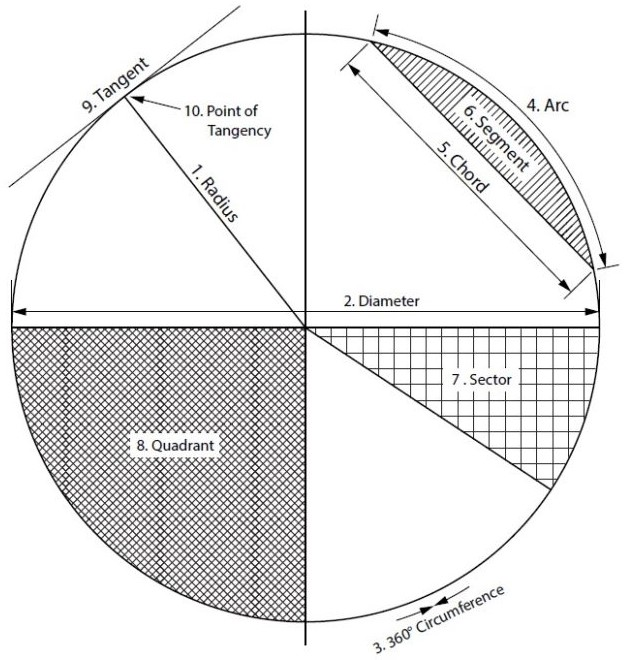# Introduction

As a sheet metal worker, we work with different lines, angles and shapes. We work with geometry. Be it in the field or in a shop, geometry is a tool we use in many different ways. From creating 2D patterns of 3D objects, to making sure roof panels are installed square, to locating duct runs and penetrations, geometry is used everyday by a sheet metal worker. This is the foundation for which all layout is done. Craftspeople who excel at this stage are able to quickly transform any complex ideas into reality.

Learning Objectives

1. Understand the process of geometric construction and its uses
2. Learn geometric terms

Geometric Terms

• Acute Angle/Trianglean angle/triangle with an angle smaller than 90°
• Bisectto divide in half
• Horizontala line/plane level with the horizon. Flat, level
• Obtuse Angle/Trianglean angle/triangle with an angle larger than 90°
• Parallela line/plane that is equal distance from another
• Parts of a Circle1. Radius – the distance from centre to any point of the circumference or half the diameter
2. Diameter – the distance across a circle at centre, twice the radius
3. Circumference – the distance around a circle, perimeter of a circle
4. Arc – a portion of a circumference
5. Chord – a straight line from 2 points on a circumference
6. Segment – the area of a circle bound by an arc and a chord
7. Sector – the area of a circle bound by 2 radii and an arc
8. Quadrant – a sector which equals one quarter of the area of a circle
9. Tangent – a line which touches only 1 point of a circumference
10. Point of Tangency – 90° to the centre of the circle
• Perpendicular – a line/plane which is 90° to another
• Right Angle/Triangle – an angle/triangle which has a 90° angle
• Vertical – a line/plane straight up and down, vertically level (plumb)
• Vertex – the point at which an angle is formed

Circle Facts:

• There are 360° in a circle.
• Circumference (or perimeter) = Pi × diameter or 2 × Pi × radius.
• Area = Pi × radius2.

# Points, Lines, Angles and Shapes

In geometry we deal with many different shapes. All shapes are made up of various points, lines and angles.

## Lines

We can define a point (A) as a single location on a shape or line.

A line (A-B) is made up of 2 points. There are different types of lines:

• horizontal (level with the horizon),
• vertical (up and down, also known as plumb),
• tilted (neither vertical nor horizontal), and
• arcs (a line from a radius point).

We also have lines which are parallel or equal distance apart and lines which are perpendicular or at right angles to one another.

## Angles

An angle is formed at a point at where 2 lines meet (vertex). We deal with three different types of angles:

• Right angles are at 90° and are very important in layout,
• Obtuse angles, which are greater than 90°, and
• acute angles, which are smaller than 90°.

## Shapes

With a combination of points, lines, and angles, we start to create shapes. Triangles (A-B-C), squares or rectangles (A-B-C-D), and circles are some of the most common shapes seen in the sheet metal industry. It is a combination of points, lines, and angles that make up different shapes —or in our case, our patterns.